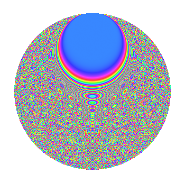# Properties

 Label 861.2.cgLevel 861 Weight 2 Character orbit cg Rep. character $$\chi_{861}(5,\cdot)$$ Character field $$\Q(\zeta_{60})$$ Dimension 1728 Newforms 1 Sturm bound 224 Trace bound 0

# Related objects

## Defining parameters

 Level: $$N$$ = $$861 = 3 \cdot 7 \cdot 41$$ Weight: $$k$$ = $$2$$ Character orbit: $$[\chi]$$ = 861.cg (of order $$60$$ and degree $$16$$) Character conductor: $$\operatorname{cond}(\chi)$$ = $$861$$ Character field: $$\Q(\zeta_{60})$$ Newforms: $$1$$ Sturm bound: $$224$$ Trace bound: $$0$$

## Dimensions

The following table gives the dimensions of various subspaces of $$M_{2}(861, [\chi])$$.

Total New Old
Modular forms 1856 1856 0
Cusp forms 1728 1728 0
Eisenstein series 128 128 0

## Trace form

 $$1728q - 24q^{3} + 196q^{4} - 32q^{7} + O(q^{10})$$ $$1728q - 24q^{3} + 196q^{4} - 32q^{7} - 36q^{10} - 36q^{12} - 8q^{15} + 196q^{16} + 18q^{18} - 48q^{19} - 20q^{21} - 88q^{22} + 6q^{24} - 196q^{25} - 68q^{28} + 2q^{30} - 36q^{31} - 180q^{33} - 120q^{36} + 12q^{37} - 10q^{39} - 24q^{40} - 104q^{42} - 80q^{43} - 66q^{45} + 80q^{46} - 40q^{49} - 48q^{51} - 60q^{52} - 120q^{54} - 80q^{57} + 8q^{58} + 138q^{60} - 60q^{61} - 30q^{63} - 384q^{64} - 150q^{66} - 8q^{67} - 24q^{70} - 50q^{72} + 108q^{75} + 96q^{78} - 24q^{79} + 40q^{81} - 312q^{82} - 40q^{84} + 8q^{85} - 30q^{87} + 112q^{88} + 84q^{93} - 168q^{94} - 24q^{96} + 64q^{99} + O(q^{100})$$

## Decomposition of $$S_{2}^{\mathrm{new}}(861, [\chi])$$ into irreducible Hecke orbits

Label Dim. $$A$$ Field CM Traces $q$-expansion
$$a_2$$ $$a_3$$ $$a_5$$ $$a_7$$
861.2.cg.a $$1728$$ $$6.875$$ None $$0$$ $$-24$$ $$0$$ $$-32$$